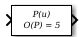# Polynomial

Perform evaluation of polynomial coefficients on input values

•Libraries:

## Description

The Polynomial block evaluates `P(u)` at each time step for the input u. You define a set of polynomial coefficients in the form that the MATLAB® `polyval` command accepts.

## Ports

### Input

expand all

Value at which to evaluate the polynomial P(u).

Data Types: `single` | `double`

### Output

expand all

Value of the polynomial P(u) evaluated at the input signal.

Data Types: `single` | `double`

## Parameters

expand all

Specify polynomial coefficients in MATLAB `polyval` form. The first coefficient corresponds to xN and the remaining coefficients correspond to decreasing orders of x. The last coefficient represents the constant for the polynomial.

#### Programmatic Use

 Block Parameter: `coefs` Type: real array Default: ```[ +2.081618890e-019, -1.441693666e-014, +4.719686976e-010, -8.536869453e-006, +1.621573104e-001, -8.087801117e+001 ]```

## Block Characteristics

 Data Types `double` | `single` Direct Feedthrough `yes` Multidimensional Signals `no` Variable-Size Signals `no` Zero-Crossing Detection `no`

## Version History

Introduced before R2006a